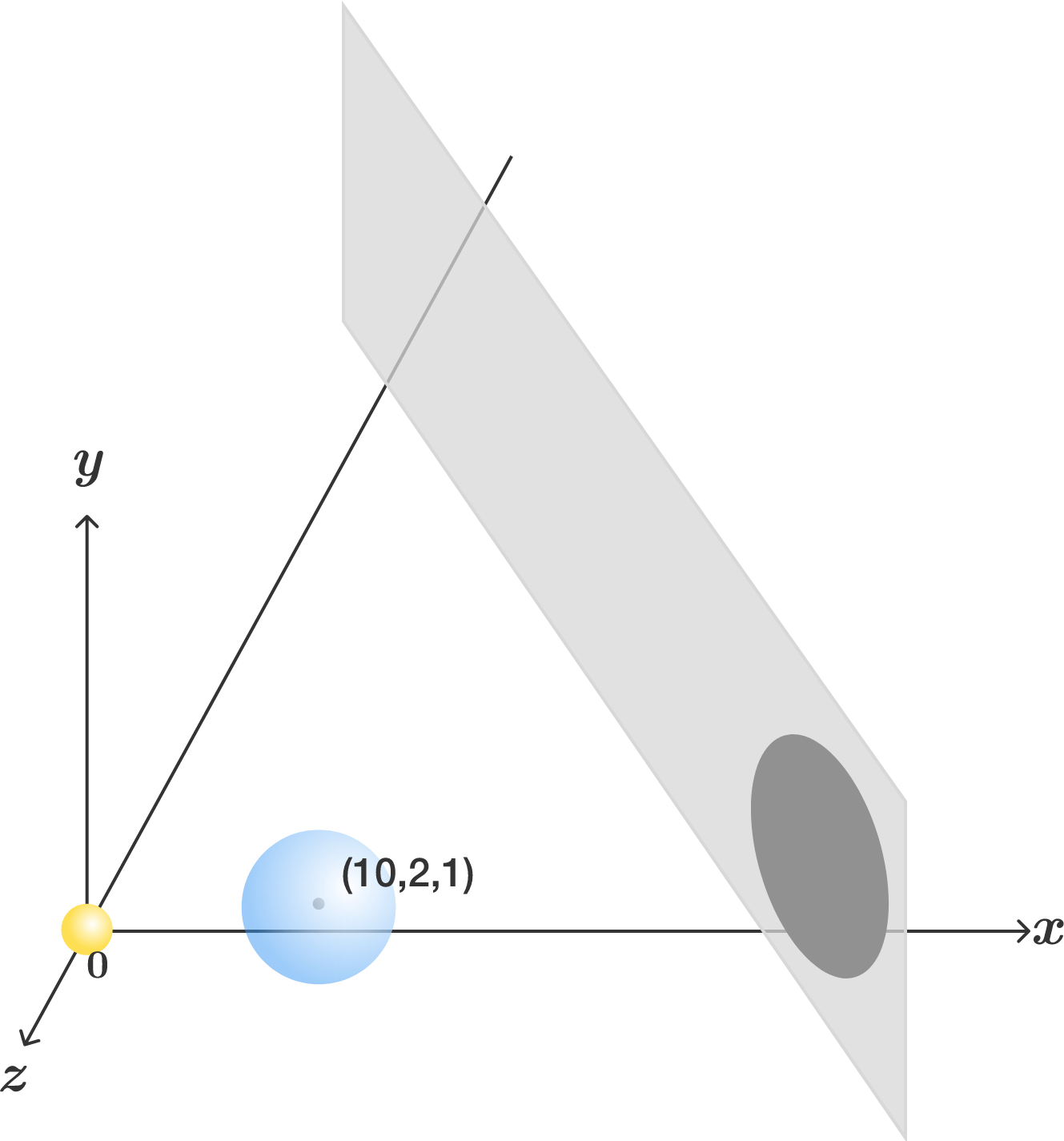Geometry Level 4

In a Cartesian coordinate system, there are 2 spheres and 1 plane described as follows:

• $S_1:$ Centered at the origin with radius 1.
• $S_2:$ Centered at $(10,2,1)$ with radius 3.
• $P:$ Defined by the equation $9x-10z=260.$

The first sphere $S_1$ acts as a light source, the second sphere $S_2$ is opaque, and the plane $P$ is a screen.

What is the area of the umbra (the darkest part of the shadow, where an observer cannot see the light source at all) on the screen?×